# Measuring Closed Figures & 3D Shapes Chapter Exam

Exam Instructions:

Choose your answers to the questions and click 'Next' to see the next set of questions. You can skip questions if you would like and come back to them later with the yellow "Go To First Skipped Question" button. When you have completed the practice exam, a green submit button will appear. Click it to see your results. Good luck!

### Page 3

#### Question 11 11. What is the area of the pictured triangle?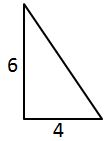#### Question 13 13. The volume of this square pyramid is 6250 cubic feet. If each side of the base is 25 feet long, what is its height?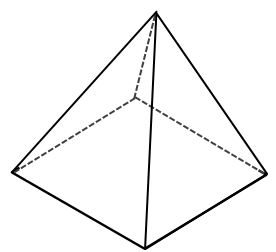#### Question 14 14. What is the volume of this cube?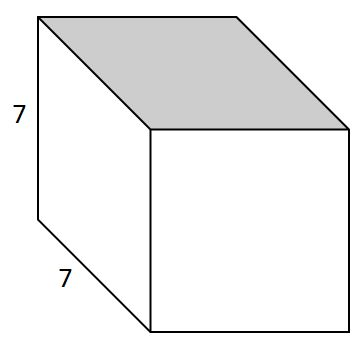### Page 4

#### Question 17 17. Find the perimeter.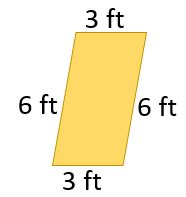#### Question 18 18. Find the perimeter.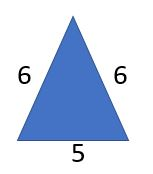### Page 5

#### Question 21 21. What is the measurement of the missing bottom side?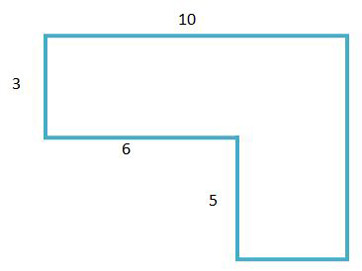#### Question 22 22. What is the perimeter?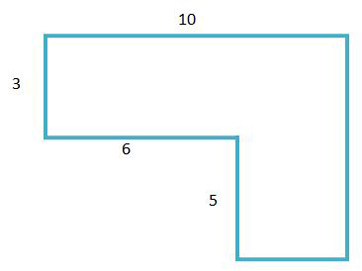#### Question 23 23. Find the area of the red area if the length of the rectangle is 13 cm, the width of the rectangle is 10 cm, and the radius of the circle is 5 cm.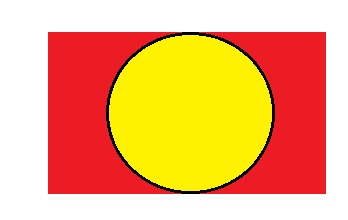### Page 6

#### Question 28 28. What is the area of ABCDE, as shown in the diagram?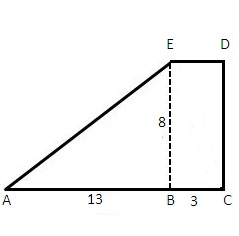#### Question 29 29. A triangular pyramid is pictured. If the height of the pyramid is 16 and the base dimensions are as shown, what is the volume?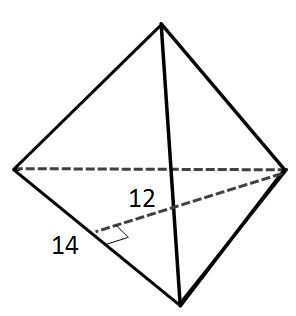#### Measuring Closed Figures & 3D Shapes Chapter Exam Instructions

Choose your answers to the questions and click 'Next' to see the next set of questions. You can skip questions if you would like and come back to them later with the yellow "Go To First Skipped Question" button. When you have completed the practice exam, a green submit button will appear. Click it to see your results. Good luck!

Support